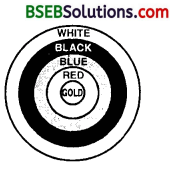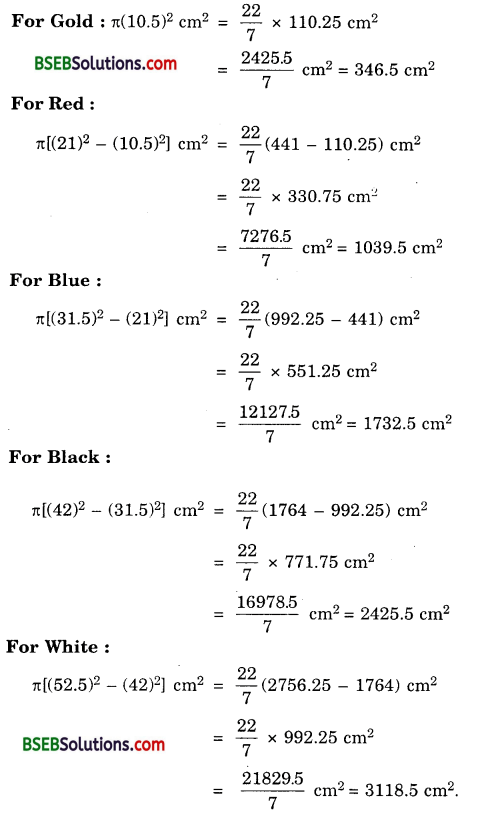# HSSlive: Plus One & Plus Two Notes & Solutions for Kerala State Board

## BSEB Class 10 Maths Chapter 12 Areas Related to Circles Ex 12.1 Textbook Solutions PDF: Download Bihar Board STD 10th Maths Chapter 12 Areas Related to Circles Ex 12.1 Book AnswersBSEB Class 10 Maths Chapter 12 Areas Related to Circles Ex 12.1 Textbook Solutions PDF: Download Bihar Board STD 10th Maths Chapter 12 Areas Related to Circles Ex 12.1 Book Answers

BSEB Class 10th Maths Chapter 12 Areas Related to Circles Ex 12.1 Textbooks Solutions and answers for students are now available in pdf format. Bihar Board Class 10th Maths Chapter 12 Areas Related to Circles Ex 12.1 Book answers and solutions are one of the most important study materials for any student. The Bihar Board Class 10th Maths Chapter 12 Areas Related to Circles Ex 12.1 books are published by the Bihar Board Publishers. These Bihar Board Class 10th Maths Chapter 12 Areas Related to Circles Ex 12.1 textbooks are prepared by a group of expert faculty members. Students can download these BSEB STD 10th Maths Chapter 12 Areas Related to Circles Ex 12.1 book solutions pdf online from this page.

## Bihar Board Class 10th Maths Chapter 12 Areas Related to Circles Ex 12.1 Books Solutions

 Board BSEB Materials Textbook Solutions/Guide Format DOC/PDF Class 10th Subject Maths Chapter 12 Areas Related to Circles Ex 12.1 Chapters All Provider Hsslive

## How to download Bihar Board Class 10th Maths Chapter 12 Areas Related to Circles Ex 12.1 Textbook Solutions Answers PDF Online?

2. Click on the Bihar Board Class 10th Maths Chapter 12 Areas Related to Circles Ex 12.1 Answers.
3. Look for your Bihar Board STD 10th Maths Chapter 12 Areas Related to Circles Ex 12.1 Textbooks PDF.
4. Now download or read the Bihar Board Class 10th Maths Chapter 12 Areas Related to Circles Ex 12.1 Textbook Solutions for PDF Free.

## BSEB Class 10th Maths Chapter 12 Areas Related to Circles Ex 12.1 Textbooks Solutions with Answer PDF Download

Find below the list of all BSEB Class 10th Maths Chapter 12 Areas Related to Circles Ex 12.1 Textbook Solutions for PDF’s for you to download and prepare for the upcoming exams:

## BSEB Bihar Board Class 10th Maths Solutions Chapter 12 Areas Related to Circles Ex 12.1

Question 1.
The radii of two circles are 19 cm and 9 cm respectively. Find the radius of the circle which has circumference equal to the sum of the circumferences of the two circles.
Solution:
Let r be the radius of the circle whose circumference is equal to the sum of the circumferences of the two circles of radii 19 cm and 9 cm.
∴ 2πr = 2π( 19) + 2π(9)
or r = 19 + 9 = 28
Hence, the radius of the new circle is 28 cm.

Question 2.
The radii of two circles are 8 cm and 6 cm respectively. Find the radius of the circle having area equal to the sum of the areas of the two circles.
Solution:
Let r be the radius of the circle whose area is equal to the sum of the areas of the circles of radii 8 cm and 6 cm.
∴ π² = π(8)² + π(6)² or r² = 8² + 6²
or r² = 64 + 36 or r² = 100
or r = 100‾‾‾‾√ = 10
Hence, the radius of the new circle is 10 cm.

Question 3.
Figure depicts an archery target marked with its five scoring areas from the centre outwards as Gold, Red, Blue, Black and White. The diameter of the region representing Gold score is 21 cm and each of the other bands is 10.5 cm wide. Find the area of each of the five scoring regions.Solution:
The area of each of five scoring regions are as under :Question 4.
The wheels of a car are of diameter 80 cm each. How many complete revolutions does each wheel make in 10 minutes when the car is travelling at a speed of 66 km per hour?
Solution:
Distance covered by the car in 10 minutes
= (66×1000×100×1060) cm
= 66000006 = 1100000 cm
Circumference of each wheel of the car
= 2πr = (2×227×40)cm [∴ r = 802 cm = 40]
Number of revolutions in 10 minutes when the car is travelling at a speed of 66 km/h = 1100000 ÷ (2 x 225 x 40)
= 4375
Hence, each wheel of car makes 4375 revolutions in 10 minutes.

Question 5.
Tick the correct answer in the following and justify your choice : If the perimeter and the area of a circle are numerically equal, then the radius of the circle is
(A) 2 units
(B) it units
(C) 4 units
(D) 7 units
Solution:
(A) Because,
Here, 2πr = πr², where r is the radius.
So, r² – 2r = 0 or r(r – 2) = 0
or r = 0 or r = 2
But, r ≠ 0. r = 2 units

## Bihar Board Class 10th Maths Chapter 12 Areas Related to Circles Ex 12.1 Textbooks for Exam Preparations

Bihar Board Class 10th Maths Chapter 12 Areas Related to Circles Ex 12.1 Textbook Solutions can be of great help in your Bihar Board Class 10th Maths Chapter 12 Areas Related to Circles Ex 12.1 exam preparation. The BSEB STD 10th Maths Chapter 12 Areas Related to Circles Ex 12.1 Textbooks study material, used with the English medium textbooks, can help you complete the entire Class 10th Maths Chapter 12 Areas Related to Circles Ex 12.1 Books State Board syllabus with maximum efficiency.

## FAQs Regarding Bihar Board Class 10th Maths Chapter 12 Areas Related to Circles Ex 12.1 Textbook Solutions

#### How to get BSEB Class 10th Maths Chapter 12 Areas Related to Circles Ex 12.1 Textbook Answers??

Students can download the Bihar Board Class 10 Maths Chapter 12 Areas Related to Circles Ex 12.1 Answers PDF from the links provided above.

#### Can we get a Bihar Board Book PDF for all Classes?

Yes you can get Bihar Board Text Book PDF for all classes using the links provided in the above article.

## Important Terms

Bihar Board Class 10th Maths Chapter 12 Areas Related to Circles Ex 12.1, BSEB Class 10th Maths Chapter 12 Areas Related to Circles Ex 12.1 Textbooks, Bihar Board Class 10th Maths Chapter 12 Areas Related to Circles Ex 12.1, Bihar Board Class 10th Maths Chapter 12 Areas Related to Circles Ex 12.1 Textbook solutions, BSEB Class 10th Maths Chapter 12 Areas Related to Circles Ex 12.1 Textbooks Solutions, Bihar Board STD 10th Maths Chapter 12 Areas Related to Circles Ex 12.1, BSEB STD 10th Maths Chapter 12 Areas Related to Circles Ex 12.1 Textbooks, Bihar Board STD 10th Maths Chapter 12 Areas Related to Circles Ex 12.1, Bihar Board STD 10th Maths Chapter 12 Areas Related to Circles Ex 12.1 Textbook solutions, BSEB STD 10th Maths Chapter 12 Areas Related to Circles Ex 12.1 Textbooks Solutions,
Share: